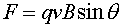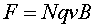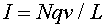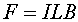Magnetic fields and forces A particle of charge q moving with velocity v through a magnetic field B feels a forcewhere q is the angle between the direction of the magnetic field and the direction of the velocity. The direction of the force is chosen perpendicular to both the velocity and magnetic field. See the right-hand-rule page. When a particle moves parallel to the magnetic field it feels no force. The magnetic field is given in units of Tesla. 1.0 T = 1.0 N·s/(mC). Other units of magnetic field are Gauss. 104 Gauss = 1.0 T. A magnetic field on the order of a Tesla is a large field which needs expensive magnets. Most cheap magnets have field on the order of 10-3 Tesla. A current-carrying wire has moving charge, therefore a segment of wire also feels a force. If a segment of wire of length L has N charges of size q moving with velocity v, perpendicular to the field, the force isThe number of charges moving by a certain point of the wire per time is the currentThis equation has the right feel as the current increases when there are more or bigger charges, if they move faster, and decreases if they are spread out over a longer length. Putting these last two equations together yieldsThus the force per unit length felt by a segment of wire in a magnetic field is the current times the magnetic field. This is easily seen with a demonstration.